# Barrel 4

Barrel of water weighs 63 kg. After off 75% water, the weight of the barrel with water is 21 kg. How many kg weigh empty barrel and how many kgs water in it?

x =  7 kg
y =  56 kg

### Step-by-step explanation:

x+y=63
x+(1-75/100)y=21

x+y=63
x+(1-75/100)•y=21

x+y = 63
100x+25y = 2100

x = 7
y = 56

Our linear equations calculator calculates it.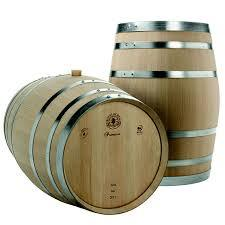Did you find an error or inaccuracy? Feel free to write us. Thank you!Tips to related online calculators
Do you have a system of equations and looking for calculator system of linear equations?

## Related math problems and questions:

• The water barrel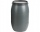The water barrel weighs 122 kg. If we pour 75% of the water out of it, it will weigh 35 kg. What is the weight of the barrel?
• Barrel 2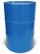Empty barrel weighs 7 kg. When it filled with water up to 45% height, weighs 60 kg. How heavy is barrel full of water?
• Barrel 3Barrel with water weights 118 kg. When we get off 75% of water, it weights 35 kg. How many kg has an empty barrel?
• Water containerContainer with water weighs 1.48 kg. When we cast 75% of water container of water weight 0.73 kg. How heavy is an empty container?
• Container with water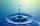The weight of a container with water is 2.48 kg. If the cast 75% water, the container with water has a weight of 0.98 kg. Determine the weight of the empty container. How much water was originally in the container?
• Barrel with liquid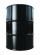Barrel with grain weight 297 kg. When it shed 48% of grain, ha weight 174 kg. What is the weight of empty barrel?
• Barrel with water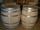The water barrel has a weight of 52 kg. When we pour a quarter of the water, the weight of the barrel is reduced to 40 kg. Determine the weight of the empty barrel.
• Barrel of oilBarrel of oil weighs 283 kg. When it mold 26% oil, weighed 216 kg. What is the mass of the empty barrel?
• The barrel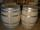The barrel is 135 cm high. If we fill it to a height of 60 cm and it weighs 163 kg. If we fill it to a height of 80 cm, it weighs 213 kg. How many kg weighs a full and how much empty barrel?
• Watering can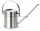A watering can full of water weighs 11 kg, the weight of water is 10 kg greater than the weight of an empty watering can, how much is the weight of the watering can?
• Camel and water84% of the camel's weight is water. After drinking, its weight increased to 832 kg, and water accounted for 85% of its weight. How much did it weigh before drinking?
• Cargo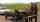The weight of the cargo car is 761 kg. The weight of the car is 23% of the total weight of car+cargo. What is the weight of the cargo load?
• Water wessel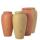The vessel containing water has a weight 19 kg. After pouring half the water its weigth 11 kg. What is the weight of the empty vessel?
• Three friendsDanica, Lenka, and Dalibor have 96 kg altogether. Lenka weighs 75% more than Dalibor, and Danica weighs 6 kg more than Dalibor. Determine the weight of Danice, Lenka, and Dalibor.
• Alcohol 2Two types of alcohol one 63% and second 75% give 20 liters of 69% alcohol. How many liters of each type are in the mixture?
• BucketThe bucket half filled with water weighs 5.55 kg, the full bucket weighs 9.85 kg. How much does the bucket weigh?
• Reservoir + waterThe reservoir filled with water weighs 12 kg and after pouring off three-quarters of the water weights 3 kg. Calculate the weight and volume of the reservoir.